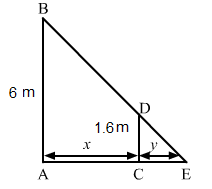# A man 160 cm tall,

Question:

A man 160 cm tall, walks away from a source of light situated at the top of a pole 6 m high, at the rate of 1.1 m/sec. How fast is the length of his shadow increasing when he is 1 m away from the pole?

Solution:

Let AB be the lamp post. Suppose at any time t, the man CD is at a distance of x km from the lamp post and y m is the length of his shadow CE.Since triangles $A B E$ and $C D E$ are similar,

$\frac{A B}{C D}=\frac{A E}{C E}$

$\Rightarrow \frac{6}{1.6}=\frac{x+y}{y}$

$\Rightarrow \frac{x}{y}=\frac{6}{1.6}-1$

$\Rightarrow \frac{x}{y}=\frac{4.4}{1.6}$

$\Rightarrow y=\frac{16}{44} x$

$\Rightarrow \frac{d y}{d t}=\frac{16}{44}\left(\frac{d x}{d t}\right)$

$\Rightarrow \frac{d y}{d t}=\frac{16}{44} \times 1.1$     $\left(\because \frac{d x}{d t}=1.1\right)$

$\Rightarrow \frac{d y}{d t}=0.4 \mathrm{~m} / \mathrm{sec}$